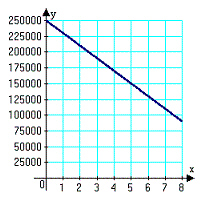Name:    Chapter 4 Post Test

Multiple Choice
Identify the choice that best completes the statement or answers the question.

1.

Which label corresponds to the coordinates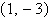?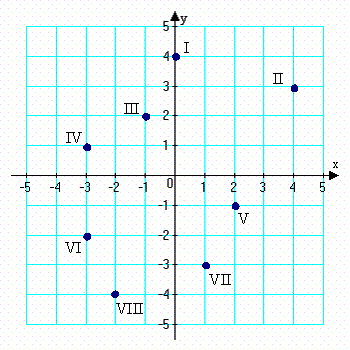a. IV b. V c. VI d. none e. VII

2.

Which label corresponds to the coordinates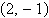?a. II b. IV c. none d. III e. V

3.

Plot the points below whose coordinates are given on a Cartesian coordinate system.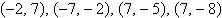a.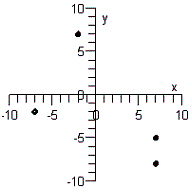d.b.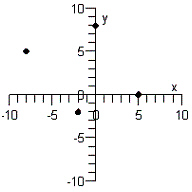e.c.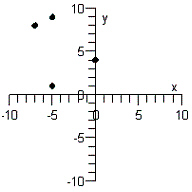4.

Plot the points below whose coordinates are given on a Cartesian coordinate system.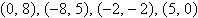a.d.b.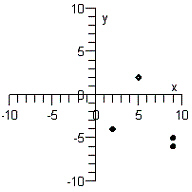e.c.5.

Find the distance between the two points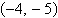and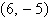.
 a. 16 b. 20 c. 24 d. 10 e. 11

6.

Find the distance between the two pointsand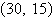.
 a. 78 b. 52 c. 49 d. 23 e. 26

7.

Find the distance between the two points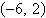and.
 a. 153 b. 102 c. 99 d. 48 e. 51

8.

Given the points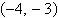and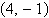Find a third point so that the three points form the vertices of a right triangle.
 a.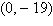b.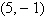c.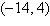d.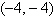e.9.

Given the points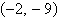andFind a third point so that the three points form the vertices of a right triangle.
 a.b.c.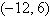d.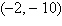e.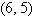10.

Find the midpoint between the two points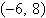and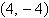.
 a.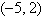b.c.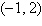d.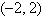e.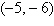11.

Find the midpoint between the two pointsand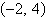.
 a.b.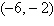c.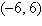d.e.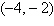12.

Find the x- and y-intercepts of the graph of the equation.
 a. x-intercept:y-intercept: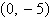b. x-intercept:y-intercept:c. x-intercept: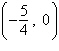y-intercept: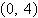d. x-intercept:y-intercept: none e. x-intercept: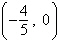y-intercept:13.

Find the x- and y-intercepts of the graph of the equation.
 a. x-intercept: (49, 0)y-intercept: (0, 7) b. x-intercept: (49, 0)y-intercept: (0, 49) c. x-intercept: (7, 0)y-intercept: (0, 49) d. x-intercept: (7, 0)y-intercept: (0, –7) e. x-intercept: (–7, 0)y-intercept: (0, –49)

14.

Find the x- and y-intercepts of the graph of the equation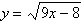.
 a. x-intercept: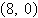y-intercept: none b. x-intercept: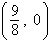y-intercept: none c. x-intercept:y-intercept: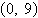d. x-intercept: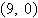y-intercept: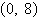e. x-intercept: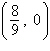y-intercept: none

15.

You purchase a jet ski for \$10,000. The depreciated value, y, after x years is given by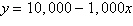. Sketch the graph of the equation given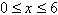.
 a.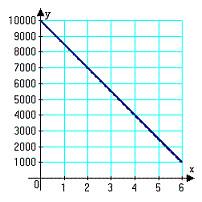d.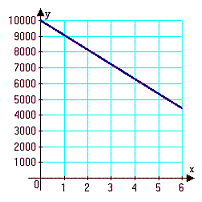b.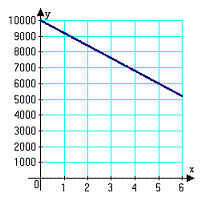e.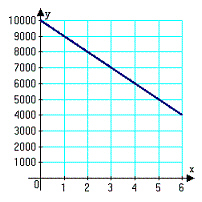c.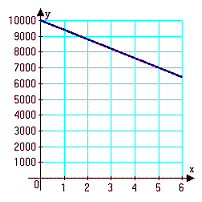16.

You purchase a house for \$250,000. The depreciated value, y, after x years is given by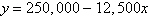. Sketch the graph of the equation given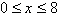.
 a.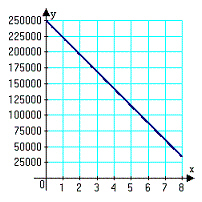d.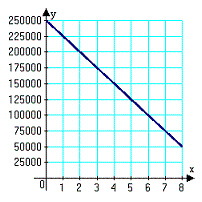b.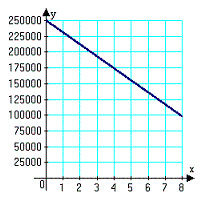e.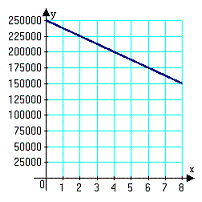c.